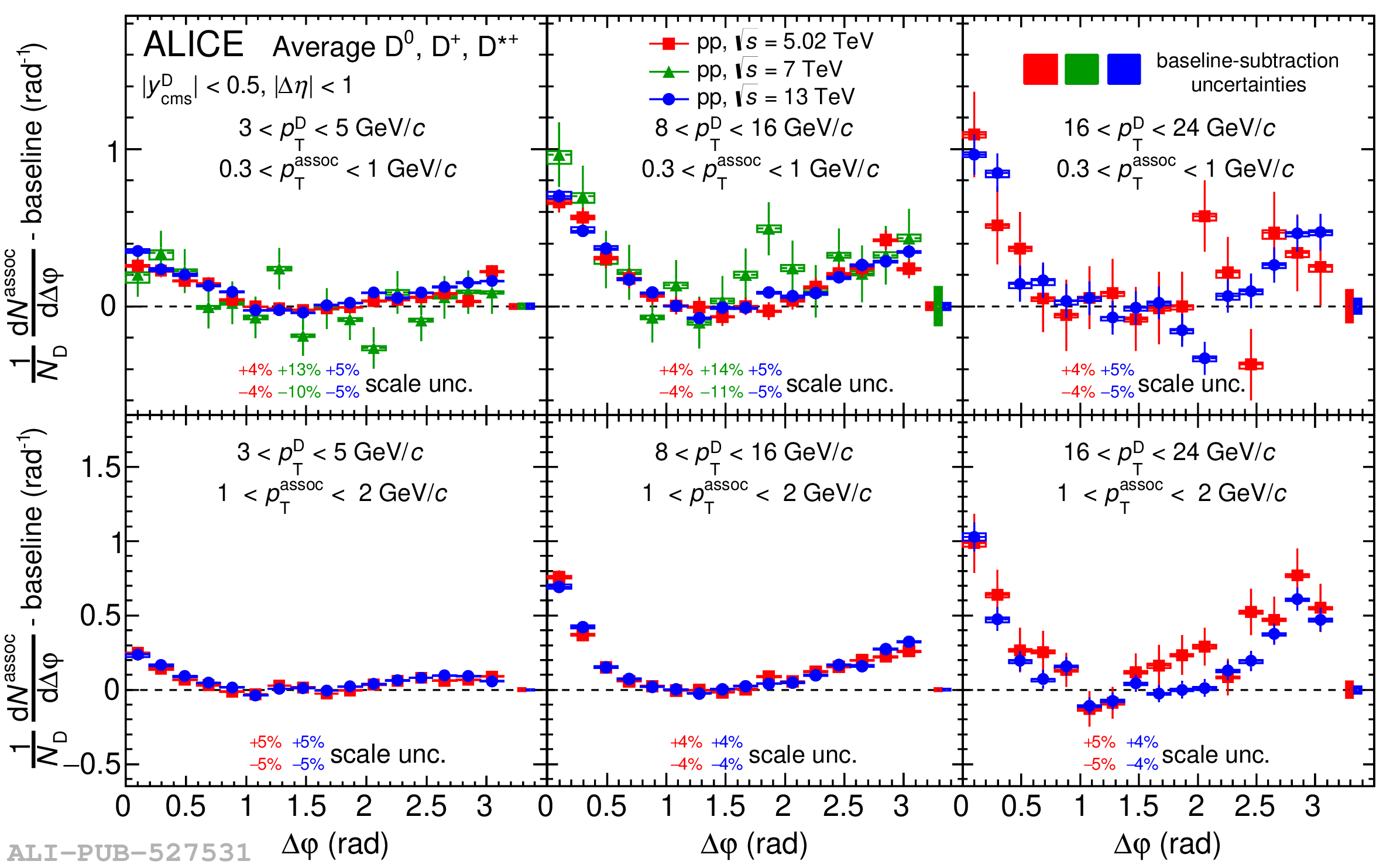# Figure 1

 Average of the azimuthal-correlation functions of $\Dzero$, $\Dplus$, and $\Dstar$ mesons with associated particles, after the baseline subtraction, in pp collisions at $\sqrt{s}$ = 5.02 , 7 , and 13 TeV, for 3 $< \ptD <$ 5 \GeVc, 8 $< \ptD <$ 16 \GeVc, and 16 $<\ptD<$ 24 \GeVc (from left to right) and 0.3 $<\ptass<$ 1 \GeVc, 1 $<\ptass<$ 2 \GeVc (top and bottom panels, respectively). Data at $\sqrt{s}$ = 7 TeV are not available for all the $p_{\mathrm{T}}$ regions. Statistical and $\Delta \varphi$-dependent systematic uncertainties are shown as vertical error bars and boxes, respectively, and $\Delta \varphi$-independent uncertainties are written as text. The uncertainties from the subtraction of the baseline are displayed as boxes at $\Delta \varphi>\pi$.